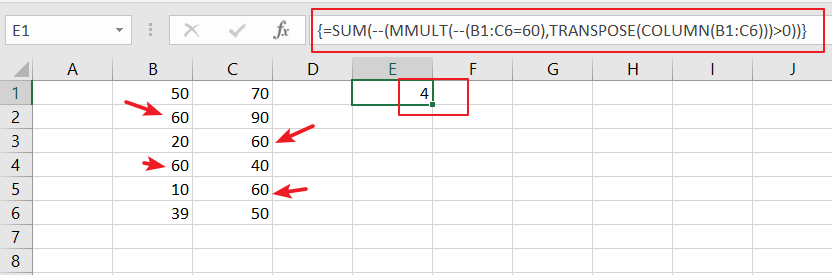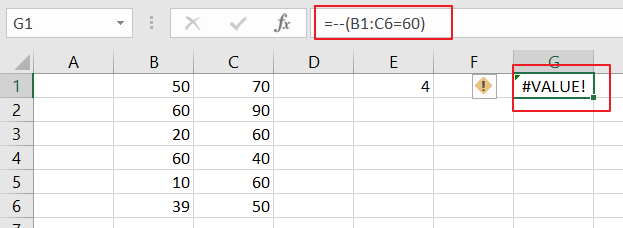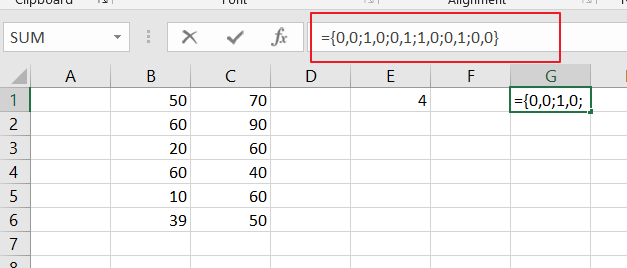# How to Count Row That Contain Specific Value in Excel

This post will guide you how to count rows that contain a specific text or number in Excel 2013/2016 or Excel office 365. How do I count the number of rows that contain a particular value while working with your current worksheet in Excel. And you can do this by a array formula based on the SUM function, the MMULT function, the TRANSPOSE function and the COLUMN function in Excel.

## General Formula:

The below general formula can help you to count rows that have specific values in Excel. Like this:

`=SUM(--(MMULT(--(criteria), TRANSPOSE(COLUMN(Range)))>0))`

Note: this formula is an array formula and you have to press “`CTRL + SHITF +Enter`” to change it from a normal formula to a array formula.

## Count Row That Contain Specific Value

Assuming that you have a data of list in range B2:C6. And you want to know the number of rows that have a particular value 60 in your given range of cells.  And you can refer to the above generic formula to create a new array formula. Like this:

`=SUM(--(MMULT(--(B1:C6=60),TRANSPOSE(COLUMN(B1:C6)))>0))`Let’s See That How This Formula Works:

`=--(B2:C6=60)`This formula is a logic criteria, and it is used to generate a TRUE and FALSE array result, and the double negative operator can be used to force the TRUE and FALSE values to 1 and 0 respectively.

`{0,0;1,0;0,1;1,0;0,1;0,0}`The Column function can be used to get the column number in an array format. And the TRANSPOSE function is used to change the column array format to row array.

Finally, the SUM function will count all those rows that have your specific value in the given range of cells.

Related Functions

• Excel COLUMN function
The Excel COLUMN function returns the first column number of the given cell reference.The syntax of the COLUMN function is as below:=COLUMN ([reference])….
• Excel SUM function
The Excel SUM function will adds all numbers in a range of cells and returns the sum of these values. You can add individual values, cell references or ranges in excel.The syntax of the SUM function is as below:= SUM(number1,[number2],…)…
• Excel TRANSPOSE function
Excel TRANSPOSE formula allows you to rotate (swap) values from rows to columns and vice versa in Excel.The Excel TRANSPOSE Function syntax:=TRANSLATE (range) …